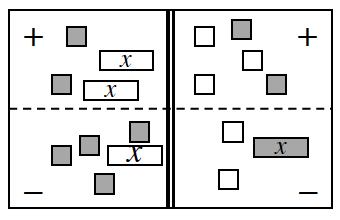### Home > CCA > Chapter A > Lesson A.1.9 > ProblemA-92

A-92.

Translate the Equation Mat at right into an equation. Remember that the double line represents “equals.” Homework Help ✎Tackle the Equation Mat corner by corner: top left, bottom left, top right, bottom right.
Remember, the problem does not ask you to simplify.

$\left(−2x + 2\right) − \left(−x + 4\right) = ?$

Use the Algebra tiles in the eTools below to help you write an equation.
Click on the link at right for the full eTool version: CCA A-92 HW eTool